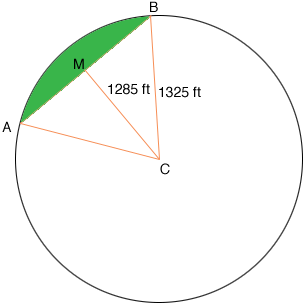Quandaries and Queries Who is asking: Other Level: All Question: A math question concerning the area asked by Staci bounded by the chord of circle and the arc was answered by Harley with the following formula: Area (S) = 1/2 (r2)theta where r2 is the radius of the circle squared where r = radius of the circle and theta is the angle in radians formed by the chord joined at its ends by radial lines. A friend of mine is a farmer and uses Pivots to irrigate portions of his land. The crop rows are in straight lines that all form chords of a large circle. The intent is to determine area between any two "boundary" rows expressed in acres. I attempted to apply the above formula using the following numbers Circle radius = 1325 feet Perpendicular distance from circle center to the chord is 1285 feet theta was calculated to be 1.13091 (using the 1285 as one side of a right triangle, solving for its angle and then doubling the result to get theta) Using the above formula, the acreage was calculated to be 22.78987374 (43560 square feet = one acre) Since an annulus of a circle bounded between radii of 1285 and 1325 feet was earlier calculated to be 7.52 acres, the above result seems inconsistent. Please advise what I need to do to get a good result in case you do not find my answer to be correct. (note: computing intermediate rows would be accomplished by subtracting the area of the "outer" section from an overall calculation that includes the outer and a larger inner section) Thanks, Chuck Hi Chuck, I don't have clear in my mind what you mean by the area between two boundary rows so let me know if I am not finding the correct area. Here is the diagram I have.M is the midpoint of the chord BA so the angle BMC is a right angle. Let the angle ACB be t radians then |MC|/|CB| = cos(t/2) and hence t = 2cos-1(1285/1325) = 0.4927 radians Hence the area of the sector CAB is 1/2r2t = 1/2132520.4927 = 432481.44 square feet which is 9.928 acres. Since triangle BMC is a right triangle, Pythagoras' theorem tells us |BM|2 + 12852 = 13252 and hence |BM| = sqrt[13252 - 12852] = 323.110 feet. Hence the area of the triangle BAC is 1/2baseheight = 323.1101285 = 415196 square feet which is 9.532 acres. Thus the area of the region shaded green in the diagram is 9.928 - 9.532 = 0.396 acres Harley Go to Math Central